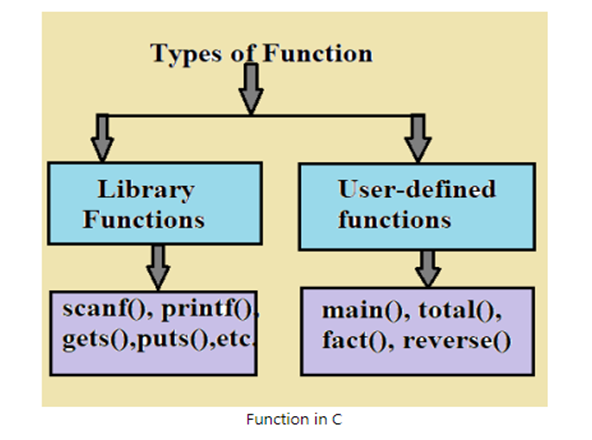# User Defined Function | User Defined Function in C

In this article User Defined Function we give the information about function is a self contained block of code that performs the particular task.

#### User Defined Function:

User Defined Function in C :

Definition

“A group of statements is known as a function.” The set of programming statements in a function is enclosed by {}. A function can be called multiple times to provide reusability and modularity to C programs.

The function is also called as procedure or subroutine in other programming languages.

In other words, “function is a self contained block of code that performs the particular task”.

Syntax:

return_type function_name(data_type parameter…)

{

// function body

}

#### Advantages of User defined function:

C functions have the following advantages.

• By using functions, we can avoid writing the same logic/code over and over again in a program.
• We can call C function from any place and at any time in any program.
• Function divided large program into many functions used for easily track a large C program
• Reusability is the main achievement of C function.
• However, calling functions in C programs is always an overhead.

#### Function Aspects:

 Sr. No. C Function Aspects Syntax 1 Function Declaration return_type  function_name(argument_list); 2 Function Call function_name(argument_list); 3 Function Definition return_type  function_name(argument_list) { Function body }

The Function declaration:

• A program must be declared globally in c program to specify the name of the function, function parameters and return type.

Function call:

• Function can be called from anywhere in the program. Parameter list should not differ in function calling and function declaration. We have to pass the same number of functions declared in the function declaration.

Function definition:

• It contains the actual statements which are to be executed. This is the most important aspect for which control comes when the function is called. Here, we should note that only one value can be returned from the function.

#### Types of function in C:1. Library Functions:

They are functions which are declared in C header files such as scanf(), printf(), gets(), puts(), ceil(), floor() etc.

1. User-defined functions:

They are the functions which are created by the C programmer so that he can use it multiple times. It reduces the complexity of a large program and optimizes the code.

#### Return Value:

A C function may or may not return a value from the function. If you don’t want to return a value from the function, use void for the return type. Let’s look at a simple example of a C function that does not return any value from the function.

Example without return value:

void hello()

{

printf(“hello c”);

}

If you want to return any value from the function, you need to use any data type such as int, long, char, etc. The return type depends on the value to be returned from the function.

Let’s look at a simple example of a C function that returns an int value from a function.

Example with return value:

int get()

{

return 100;

}

In the above example, we have to return 100 as the value, so the return type is int.

Different aspects of function calling:

A function may or may not accept any number of arguments. It may or may not return any value. Based on these facts, there are four different aspects to a function call.

#### // 1. The function with no arguments and with no return value

#include<stdio.h>

#include<conio.h>

void total();        // function declaration

void main()

{

clrscr();

total();      // function call

getch();

}

void total()       // function defination

{

int a,b;

printf(“\n Enter the two numbers: “);

scanf(“%d%d”,&a,&b);

printf(“\n\n Addition of Two numbers: %d”,a+b);

}

Output:

Enter the two numbers: 10

20

#### // 2. The function with no arguments but return a value

#include<stdio.h>

#include<conio.h>

int total();        // function declaration

void main()

{

int sum=0;

clrscr();

sum=total();      // function call

printf(“\n\n Addition of Two numbers: %d”,sum);

getch();

}

int total()       // function defination

{

int a,b;

printf(“\n Enter the two numbers: “);

scanf(“%d%d”,&a,&b);

return(a+b);

}

Output:

Enter the two numbers: 4

5

#### // 3. function with arguments but no return a value

#include<stdio.h>

#include<conio.h>

void total(int,int);        // function declaration

void main()

{

int a,b;

clrscr();

printf(“\n Enter the two numbers: “);

scanf(“%d%d”,&a,&b);

total(a,b);      // function call

getch();

}

void total(int a,int b)       // function defination

{

printf(“\n\n Addition of Two numbers: %d”,a+b);

}

Output:

Enter the two numbers: 6

6

#### // 4. function with arguments and return a value

#include<stdio.h>

#include<conio.h>

int total(int a, int b);        // function declaration

void main()

{

int a,b,sum=0;

clrscr();

printf(“\n Enter the two numbers: “);

scanf(“%d%d”,&a,&b);

sum=total(a,b);      // function call

printf(“\n\n Addition of Two numbers: %d”,sum);

getch();

}

int total(int a, int b)       // function defination

{

return(a+b);

}

Output:

Enter the two numbers: 100

200

#### Some More:

POP- Introduction to Programming Using ‘C’

DS – Data structure Using C

OOP – Object Oriented Programming

Java Programming

DBMS – Database Management System

RDBMS – Relational Database Management System